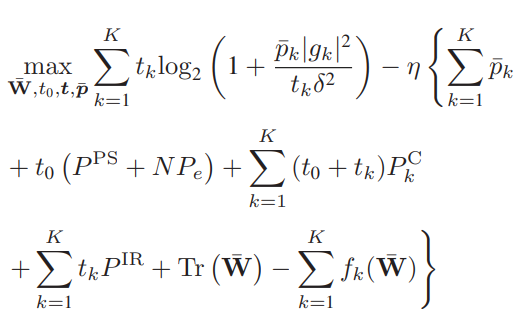# How to solve this problem {convex} ./ {real affine}

Hi,
I want to write`````` R1(k,1)=(rel_entr(t(k,1)+t(K,1)^2*delta^2/(p_p(k,1)*g(k)^2),t(K,1)^2*delta^2/(p_p(k,1)*g(k)^2))+rel_entr(t(K,1)^2*delta^2/(p_p(k,1)*g(k)^2),t(k,1)+t(K,1)^2*delta^2/(p_p(k,1)*g(k)^2)))/log(2);
``````

for the first expressions R1(K,1);

But
Disciplined convex programming error:
Cannot perform the operation: {convex} ./ {real affine}